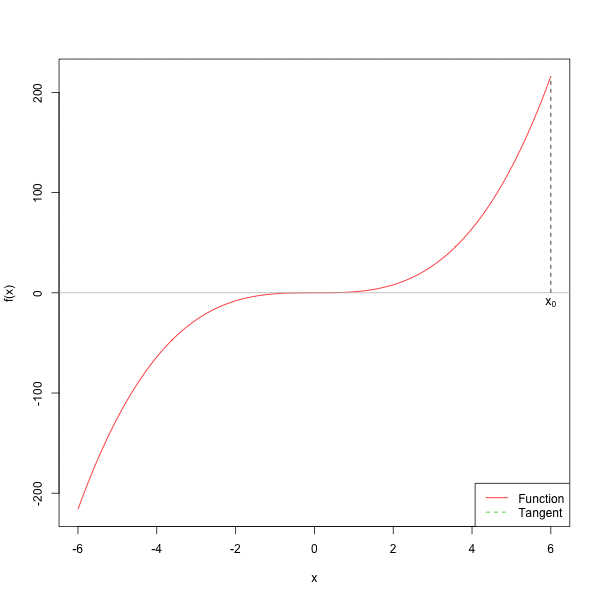# 第 2 章 求根算法

## 2.1 二分法

1. 选择初始区间 $$[a, b]$$，且 $$\text{sign}(f(a)) \neq \text{sign}(f(b))$$

2. 按照以下迭代过程进行迭代直到 $$|b-a|<\epsilon$$$$|f(b) - f(a)|<\epsilon$$

1. 计算中点 $$c = \frac{a+b}{2}$$
2. $$f(c) = 0$$，终止迭代；
3. $$\text{sign}(f(a)) \neq \text{sign}(f(c))$$，则 $$b \leftarrow c$$，否则 $$a\leftarrow c$$，返回第1步。
3. 输出 $$c$$

bisection.root <- function(f, a, b, tol = 1e-9,
max.iter = 100){
iter <- 0
if (abs(f(a)) < tol) return(a)
if (abs(f(b)) < tol) return(b)
# 当初始区间两端同号时，认为没有根
if (f(a) * f(b) > 0) return(NULL)

# 迭代直到满足条件
while((abs(b-a) > tol) && (iter < max.iter)) {
middle_point <- (a + b) / 2
iter <- iter + 1
fc = f(middle_point)
cat("迭代第", iter, "次，a =", a, "，b =", b, '\n')

# 当中点的函数值很接近0时，认为该点为根
if (abs(fc) < tol) return(middle_point)
# 当中点与左端点的符号不同时，以中点为新的右端点进行查找
if (fc * f(a) < 0){
b <- middle_point
} else {
a <- middle_point
}
}
return((b+a)/2)
}

$$[-4, 0]$$作为初始区间，可以找到其中一个根约等于$$-2.236$$，将初始区间换成$$[0,4]$$后可以找到另一个根约等于$$2.236$$

f <- function(x){x^2 - 5}
roots <- bisection.root(f, 0, 4, tol = 1e-3)
#> 迭代第 1 次，a = 0 ，b = 4
#> 迭代第 2 次，a = 2 ，b = 4
#> 迭代第 3 次，a = 2 ，b = 3
#> 迭代第 4 次，a = 2 ，b = 2.5
#> 迭代第 5 次，a = 2 ，b = 2.25
#> 迭代第 6 次，a = 2.125 ，b = 2.25
#> 迭代第 7 次，a = 2.188 ，b = 2.25
#> 迭代第 8 次，a = 2.219 ，b = 2.25
#> 迭代第 9 次，a = 2.234 ，b = 2.25
#> 迭代第 10 次，a = 2.234 ，b = 2.242
#> 迭代第 11 次，a = 2.234 ，b = 2.238
#> 迭代第 12 次，a = 2.234 ，b = 2.236当函数具有多个根时，需要调整左右端点来得到所有的根。上图中的函数 $$f(x)=x^2$$ 无法找到 $$[a,b]$$ 使得 $$\text{sign}(f(a))\neq \text{sign}(f(b))$$，但该函数具有根 $$x=0$$

## 2.2 收敛速度

### 2.2.1 线性收敛

$\lim\limits_{n\rightarrow \infty}\frac{|x_{n+1}-x_\infty|}{|x_n-x_\infty|} \leq r,$则称序列 $$\{x_n\}$$ 线性收敛。

$\frac{|x_{n+1} - x_{\infty}|}{|x_n - x_{\infty}|} = \frac{(\frac{1}{2})^{n+1}}{(\frac{1}{2})^n} = \frac{1}{2}$该序列的收敛速度为 $$\frac{1}{2} \in (0, 1)$$

### 2.2.2 超线性收敛

$\lim\limits_{n\rightarrow \infty}\frac{|x_{n+1}-x_\infty|}{|x_n-x_\infty|} = 0,$则称序列 $$\{x_n\}$$ 超线性收敛, 相比于线性收敛，超线性收敛的速度更快。线性收敛的序列永远不会收敛到最优值 $$x_\infty$$，而超线性收敛可以收敛到最优值。

$\lim_{n\rightarrow\infty}\frac{|x_{n+1} - x_{\infty}|}{|x_n - x_{\infty}|} = \frac{(\frac{1}{n+1})^{n+1}}{(\frac{1}{n})^n} = 0$该序列超线性收敛。

### 2.2.3 平方收敛

$\lim\limits_{n\rightarrow \infty}\frac{|x_{n+1}-x_\infty|}{|x_n-x_\infty|^2} < M,$则称序列 $$\{x_n\}$$ 平方收敛, 相比于之前提到的线性收敛和超线性收敛，平方收敛的速度最快。

$\lim_{n\rightarrow\infty}\frac{|x_{n+1} - x_{\infty}|}{|x_n - x_{\infty}|^2} = \frac{(\frac{1}{n+1})^{2^{n+1}}}{(\frac{1}{n})^{2^{n+1}}} = (\frac{n}{n+1})^{2^{n+1}}\leq 1$该序列平方收敛。

### 2.2.4 案例：二分法的收敛速度

$\frac{|x_{n+1} - x_{\infty}|}{|x_n - x_{\infty}|} = \frac{2^{-(n+1)}|b_0-a_0|}{2^{-n}|b_0-a_0|} = \frac{1}{2},$因此二分法线性收敛。

## 2.3 牛顿法

$0 = f(x^*) = f(x_0+h) \approx f(x_0) + h f^{\prime}(x_0),$

$h \approx-\frac{f\left(x_{0}\right)}{f^{\prime}\left(x_{0}\right)}.$

$x^*=x_{0}+h \approx x_{0}-\frac{f\left(x_{0}\right)}{f^{\prime}\left(x_{0}\right)},$

$x_{1}=x_{0}-\frac{f\left(x_{0}\right)}{f^{\prime}\left(x_{0}\right)}.$

$x_{2}=x_{1}-\frac{f\left(x_{1}\right)}{f^{\prime}\left(x_{1}\right)}.$

1. 选择初始猜测点 $$x_0$$，设置 $$n = 0$$

2. 按照以下迭代过程进行迭代：

$\begin{equation} x_{n+1}=x_{n}-\frac{f\left(x_{n}\right)}{f^{\prime}\left(x_{n}\right)}. \end{equation}$

1. 计算 $$|f(x_{n+1})|$$

1. 如果 $$|f(x_{n+1})| \leq \epsilon$$，停止迭代；
2. 否则，返回第 2 步。newton.root <- function(f, x0 = 0, tol = 1e-9,
max.iter = 100) {
x <- x0
cat(paste0("初始值：x = ", x, "\n"))
fx <- ftn(f, x)
iter <-  0
# xs用来保存每步迭代得到的x值
xs <- list()
xs[[iter + 1]] <- x
# 继续迭代直到满足停止条件
while ((abs(fx$f) > tol) && (iter < max.iter)) { x <- x - fx$f/fx$fgrad fx <- ftn(f, x) iter <- iter + 1 xs[[iter + 1]] <- x cat(paste0("迭代第", iter, "次：x = ", x, "\n")) } # output depends on success of algorithm if (abs(fx$f) > tol) {
cat("算法无法收敛\n")
return(NULL)
} else {
cat("算法收敛\n")
return(xs)
}
}

ftn <- function(f, x){
df <- deriv(f, 'x', func = TRUE)
dfx <- df(x)
f <- dfx
}

f <- expression(x^2 - 5)
roots <- unlist(newton.root(f, 5))
#> 初始值：x = 5
#> 迭代第1次：x = 3
#> 迭代第2次：x = 2.33333333333333
#> 迭代第3次：x = 2.23809523809524
#> 迭代第4次：x = 2.23606889564336
#> 迭代第5次：x = 2.23606797749998
#> 算法收敛